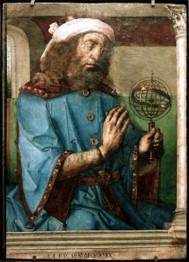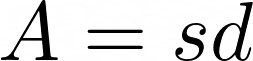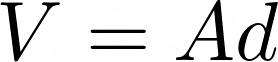## PappusPappus of Alexandria was one of the last great Greek mathematicians of antiquity, known for his Synagoge or Collection, and for Pappus’s hexagon theorem in projective geometry. Nothing is known of his life, other than what can be found in his own writings: that he had a son named Hermodorus, and was a teacher in Alexandria.

Collection, his best-known work, is a compendium of mathematics in eight volumes, the bulk of which survives. It covers a wide range of topics, including geometry, recreational mathematics, doubling the cube, polygons and polyhedra.

Pappus’ Theorems on Surfaces and Solids of Revolution

His first theorem states that the surface area A of a surface of revolution generated by rotating a plane curve C about an axis external to C and on the same plane is equal to the product of the arc length s of C and the distance d traveled by the geometric centroid of C:The second theorem states that the volume V of a solid of revolution generated by rotating a plane figure F about an external axis is equal to the product of the area A of F and the distance d traveled by the geometric centroid of F. (The centroid of F is usually different from the centroid of its boundary curve C.) That is: